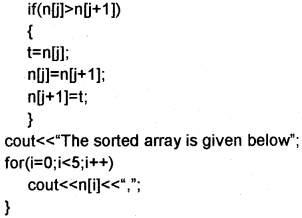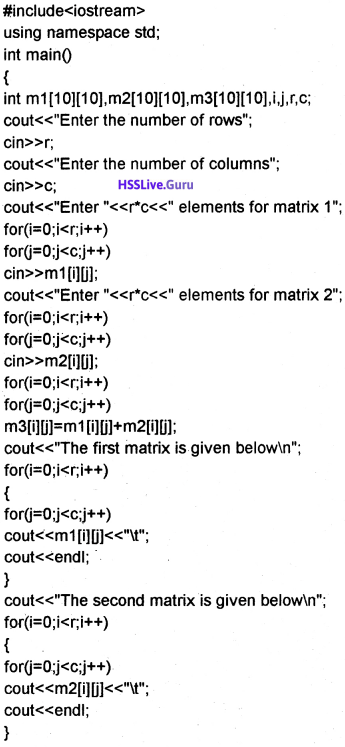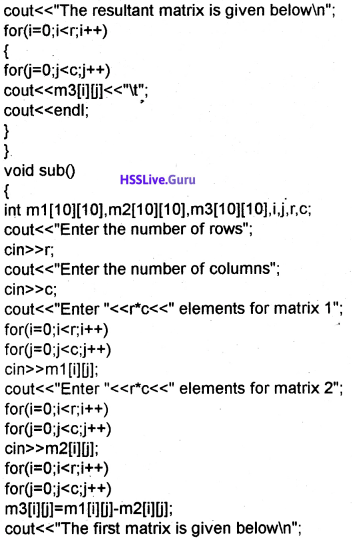# Plus One Computer Science Chapter Wise Questions and Answers Chapter 8 Arrays

Students can Download Chapter 8 Arrays Questions and Answers, Plus One Computer Science Chapter Wise Questions and Answers helps you to revise the complete Kerala State Syllabus and score more marks in your examinations.

## Kerala Plus One Computer Science Chapter Wise Questions and Answers Chapter 8 Arrays

### Plus One Arrays One Mark Questions and Answers

Question 1.
From the following which is not true for an array.
(a) It is easy to represent and manipulate array variable
(b) Array uses a compact memory structure
(c) Readability of program will be increased
(d) Array elements are dissimilar elements
(d) Array elements are dissimilar elementsQuestion 2.
Consider the following declaration.
int mark(50) Is it valid? If no give the correct declaration
It is not valid. The correct declaration is as follows, int mark. Use square brackets instead of parenthesis

Question 3.
Consider the following declaration.
int mark
The index of the last element is ________.
199

Question 4.
Consider the following declaration int mark. The index of the first element is ________.
0

Question 5.
Consider the following int age = {15,16,17,18};
From the following which type of initialisation is this.
(a) direct assignment
(b) along with variable declaration
(c) multiple assignment
(d) None of these
(b) along with variable declaration

Question 6.
From the following which is used to read and display array elements.
(a) loops
(b) if
(c) switch
(a) loops

Question 7.
Write down the corresponding memory consumption in bytes

1. int age = ——-
2. charname = ——-
3. int age= ——–

1. 2 × 10 = 20 bytbs (2 bytes for one integer)
2. 1 × 10 = 10 (one byte for each character)
3. 2 × 10 ×10 = 200 (2 × (100 elements))Question 8.
A two dimensional array is having ________ number of subscript
(a) one
(b) two
(c) three
(d) none of these
(b) two

Question 9.
Consider the following
int age;
In this array how many elements will contain.
10 × 10 = 100 elements

Question 10.
Consider the following int age = {12, 13, 14};
cout<<age;
What will be the output?
(а) 14
(b) 12
(c) 13
(d) 0
(d) 0

Question 11.
The elements of 2 dimensional array can be read using _________ loop.
nested loop

Question 12.
________ is the process of reading/visiting elements of an array.
traversalQuestion 13.
Anjali wants to read the 10 marks that already stored in an array and find the total. This process is known as ________.
(a) insertion
(b) deletion
(c) traversal
(d) linear search
(c) traversal

Question 14.
Mani wants to check a number from 100 numbers already stored in an array. This process is called ___________.
(a) insertion
(b) deletion
(c) searching
(d) None of these
(c) searching

Question 15.
Divide and conquer method used in ____________ search.
binary

Question 16.
“Each element of the array is composed with value to be searched from the beginning of the array”. This method is adopted by _____________ search.
linear search

Question 17.
____________ search requires a sorted array as input.
binary search

Question 18.
___________ searching is slower for larger array.
linear searching

Question 19.
____________ is a simple sorting algorithm.
(a) bubble sort
(b) selection sort
(c) linear search
(d) binary search
(a) bubble sort

Question 20.
Adjacent elements are checked and inter changed in _____________ sort.
bubble sort

Question 21.
The array is divided into sorted part and unsorted part in ____________ sort.
selection.Question 22.
The elements of an array of size ten are numbered from _____ to _______.
0 to 9

Question 23.
Element mark is which element of the array?
(a) The sixth
(b) the seventh
(c) the eighth
(d) impossible to tell
(b) the seventh

Question 24.
When a multidimensional array is accessed, each array index is ________.
(a) Separated by column.
(b) Surrounded by brackets and separated by commas.
(c) Separated by commas and surrounded by brackets.
(d) Surrounded by brackets,
(d) surrounded by brackets

Question 25.
You have used a 2D array with the Name Mat representing a matrix. Write the C++ expression to access the 3rd element in the 2nd row.
mat ;

Question 26.
Write a C++ statement that defines a string variable called ‘name’ that can hold a string of upto 20 characters.
char name[21j;

Question 27.
Given some array declaration. Pick the odd man out.
Float a[+40], int num[0-10], double , char name, amount of float.
char name. It is a valid array decalaration the remaining are not valid.

Question 28.
__________ is a collection of elements with same datatype.
ArrayQuestion 29.
int num;
The above C++ statement declares an array named num that can store maximum ________ integer numbers. (SEP-2015 (IMP) (1)
(а) 9
(b) 10
(c) N
(d) none of these
(b) 10

Question 30.
Declare a two dimensional array to store the elements of a matrix with order 3 × 5. (MARCH-2016) (1)
int m ; or float m 

Question 31.
_________ search method is an example for ‘divide and conquer method’. (SEP-2016) (1)
Binary

Question 32.
int AR
How many bytes will be allocated for this array? (1)
To store an integer 4 bytes needed so AR needs 10 × 4 = 40 bytes

Question 33.
Find the value of score  based on the following declaration statement. int score  = {988,87,92,79,85}; (MARCH-2017) (1)Hence (158)10 = (9E)16

### Plus One Arrays Two Mark Questions and Answers

Question 1.
Whether the statement char text[] = “COMPUTER”; is True/False? Justify.
It is a single dimensional array. If the user doesn’t specify the size the operating system allocates the number of characters + one (for null character for text) bytes of memory. So here OS allocates 9 bytes of memory.

Question 2.
Given a word ” Mathematics”. How will you locate this word in a standard dictionary. What are the steps used for this purpose
In the standard dictionary the words are arranged in sorted order so binary search method is more suitable to find the word “MATHEMATICS”.Question 3.
How can you find out the word “MATHEMATICS” from a text book. Which is the most convenient method? Justify your answer.
In a text book the words are not arranged in sorted order so linear search method is convenient.

Question 4.
Consider the statement char str[] = “PROGRAM” What will be stored in last location of this array. Justify.
The last location is the null character(\0) because each string must be appedend by a null character.

Question 5.
Explain different array operations in detail.

1. Traversal: All the elements of an array is visited and processed is called traversal
2. Search: Check whether the given element is present or not
3. Sorting: Arranging elements in an order is called sorting

Question 6.
Read the following C++ statement: int MAT;

1. How many bytes will be allocated for this array?
2. Suppose MAT is a 2D array that contains the elements of a square matrix. Write C++ statements to find the’ sum of all the elements in the array. (MARCH – 2015) (1)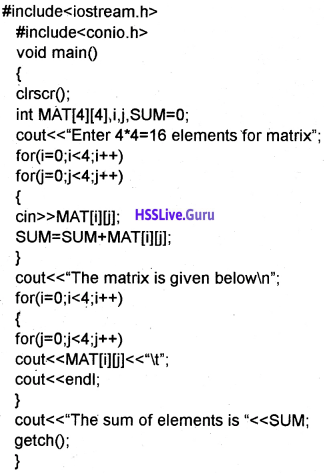Question 7.
Declare an array of size 5 and intitialize it with the numbers 8, 7, 2, 4 and 6. (MARCH-2016) (2)
int n = {8, 7, 2, 4, 6};

Question 8.
Predict the output of the following C++ program.
#include <iostream.h>
int main()
{
int array[] = {1, 2, 4, 6,7,5};
for (int n =1; n<=5; n++)
array [n] = array [n – 1 ];
for (n = 0; n <= 5; n++)
cout<< array [n];
return 0;
}
1, 1, 1, 1, 1, 1

Question 9.
Suppose M is a 2D array that contains the elements of a square matrix. Write C++ statements to find the sum of the diagonal elements. (SCERT SAMPLE – II) (2)
for (i = 0; i < 5, i++)
for (j = 0; j < 5; j++)
if (i == j)
S = S + M[i][j];

Question 10.

1. Write C++ program for sorting a list of numbers using Bubble Sort Method. (2)
2. Write the different passes of sorting the following numbers using Bubble Sort.
32, 21, 9, 17, 5

1. Example for Bubble sort is given below2. Bubble sort (ascending order):
Consider the first 2 elements of the numbers and compare it. 32 is greater than 21 then interchange both of them. Then the numbers are
21, 32, 9, 17, 5
Next compare 32 and 9. Here 32 is greater so interchange both of them. Then the numbers are
21, 9, 32, 17, 5
Next compare 32 and 7. Then interchange and the numbers are
21, 9, 17, 32, 5
Next compare 32 and 5. Then interchange and the numbers are
21, 9, 17, 5, 32.
After the first phase the largest number is on the right side. Similarly do the remaining. At last we will get the result as follows.
5, 9, 17, 21, 32Question 11.
Suppose M  is a 2D array that contains the elements of a square matrix. Write C++ statements to find the sum of the diagonal elements. (MARCH-2017)### Plus One Arrays Three Questions and Answers

Question 1.
Suppose you are given Total mark of 50 students in a class

1. How will you store these values using ordinary variable?
2. Is there any other efficient way to store these values? Give reason.

We have to declare 50 variables individually to store total marks of 50 students. It is a laborious work. Hence we use array, it is an efficient way to declare 50 variables. With a single variable name we can store multiple elements.
eg: int mark. Here we can store 50 marks. The first mark is in mark, second is in mark…. etc the fiftieth mark is in mark,

Question 2.
Four friends are in a queue for watching a movie. How can you locate a particular person from that queue? Write all the steps sequentially.
Locate a particular person from a queue can be carried out by using two ways.
1. Linear search:
In this method each element of the array is compared with the element to be searched starting from the first element. If it finds the position of the element in the array is returned.

2. Binary search:
It uses a technique called divide and conquer method. It can be performed only on sorted arrays. First we check the element with the middle element. There are 3 possibilities. The first possibility is the searched element is the middle element then search can be finished.

The second possibility is the element is less than the middle value so the upper bound is the middle element. The third possibility is the element is greater than the middle value so the lower bound is the middle element. Repeat this process.Question 3.
Total mark of 50 students in a class are given in an array. A bonus of 10 marks is awarded to all of them. Write the program code for making such a modification.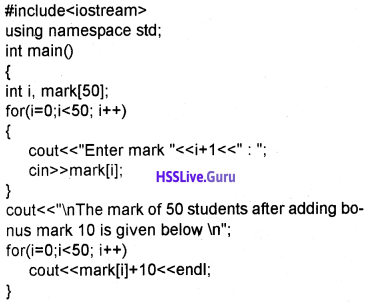Question 4.
In School Assembly, try to arrange all the students in your class according to the increasing order of their heights separately for male/female students. Smallest one will be in front and tallest one at the last position. Write sequential steps for doing this, in two different methods.
Arranging elements from smallest to largest (ascending) or largest to smallest(descending) is called sorting. There are two sorting methods
1. Bubble sort:
It is a simple sorting method. In this sorting considering two adjacent elements if it is out of order, the elements are interchanged. After the first iteration the largest(in the case of ascending sorting) or smallest(in the case of descending sorting) will be the end of the array. This process continues.

2. Selection sort:
In selection sort the array is divided into two parts, the sorted part and unsorted part. First smallest element in the unsorted part is searched and exchanged with the first element. Now there is 2 parts sorted part and unsorted part. This process continues.

Question 5.
Using the concept obtained from algorithms in question number (9), write complete program for the two types of sorting.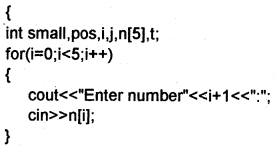Question 6.
Collect the heights of 12 students from your class in which 7 students are male and others are female students. Suppose these male and female students be seated in two separate benches and you are given a place which is used for sitting these 12 students in linear form. How will you combine and make them sit without mixing male/female students. Write a program for the same.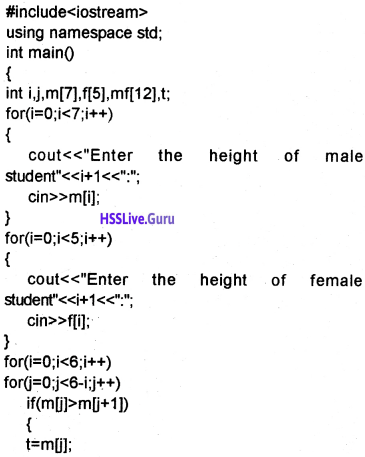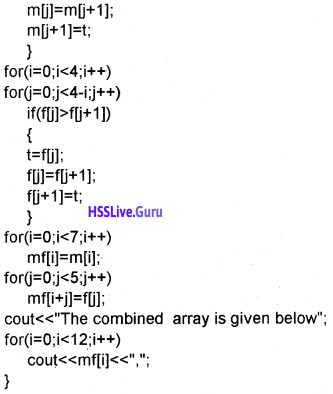Question 7.
Collect the heights of 12 students from your class in which 7 students are male and others are female students. Suppose you are given the heights according to a sorted order separately for male/female students. How will you combine these group according to the same order in linear form. Write a program for the same.Question 8.
Write the program code for counting the number of vowels from your school name.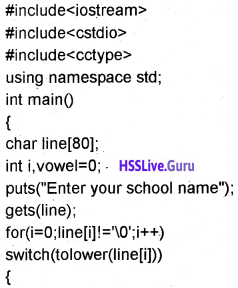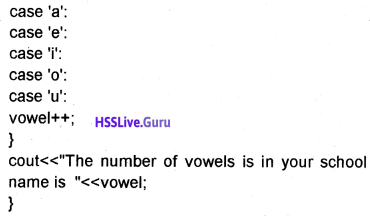Question 9.
Write the program code for counting the number of words from the given string.” Directorate of Higher Secondary Examination”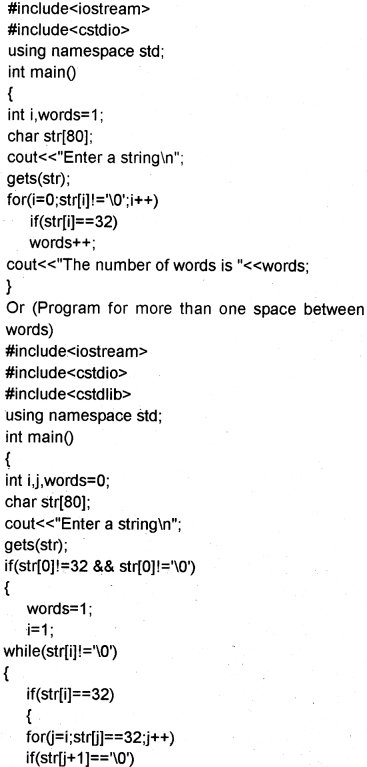Question 10.
Given a word like “ECNALUBMA” Write the program code for arranging it in into a meaningful word.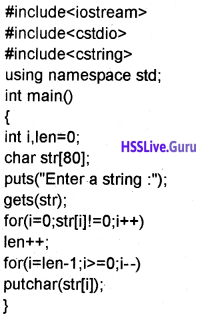Question 11.
Explain the needs for arrays Array
Array is collection of same type of elements. With the same name we can store more elements. The elements are distinguished by using its index or subscript. To store 50 marks of 50 students we have to declare 50 variables, it is a laborious work.

Hence the need for arrays arise. By using array this is very easy as follows int mark. Here the index of first element is 0, then 1, 2, 3, etc upto 49.

Question 12.
Explain different types of arrays.
There are two tyes of array
1. Single dimensional:
It contains only one index or subscript. The index starts from 0 and ends with size-1.
eg: int n;
char name;

2. Multi-dimensional:
It contains more than one index or subscript. The two dimensional array contains two indices, one for rows and another for columns. The row index starts from 0 and end at row size-1 and column index starts at 0 and ends at colunn size-1.
eg: int n can store 10 × 10 = 100 elements. The index of the first element is n and index of the 100th element is n,

Question 13.
Write a program to read the 5 marks of a students and display the marks and total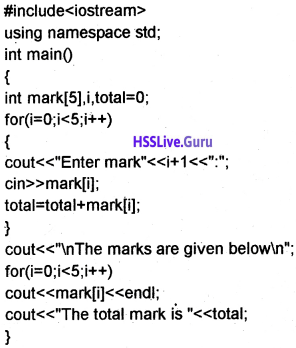Question 14.
Write a program to read 3 marks of 5 students and find the total and display it.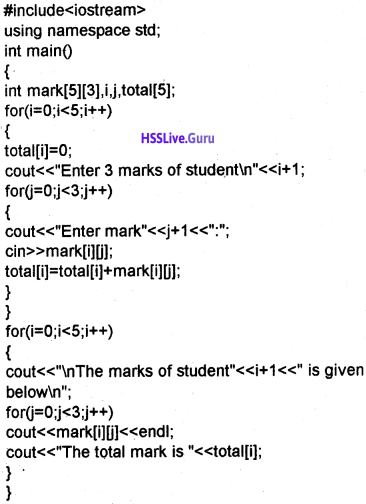Question 15.
Differentiate linear search and binary search
1. Linear search:
In this method each element of the array is compared with the element to be searched starting from the first element. If it finds the position of the element in the array is re¬turned.

2. Binary search:
It uses a technique called divide and conquer method. It can be performed only on sorted arrays. First we check the element with the middle element. There are 3 possibilities. The first possibility is the searched element is the middle element then search can be finished.

The second possibility is the element is less than the middle value so the upper bound is the middle element. The third possibility is the element is greater than the middle value so the lower bound is the middle element. Repeat this process.

Question 16.
Differentiate bubble sort and selection sort.
1. Bubble sort:
It is a simple sorting method. In this sorting considering two adjascent elements if it is out of order, the elements are interchanged. After the first iteration the largest(in the case of ascending sorting) or smallest (in the case of descending sorting) will be the end of the array. This process continues.

2. Selection sort:
In selection sort the array is divided into two parts, the sorted part and unsorted part. First smallest element in the unsorted part is searched and exchanged with the first element. Now there is 2 parts sorted part and unsorted part. This process continues.

Question 17.
Write a program to read a string and a character and find the character by using linear search.Question 18.
Write a program to read marks of 10 students and read a mark and check whether it is in the array or not using binary search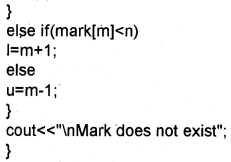Question 19.
Write a program reverse a string without using a function.Question 20.
Write a program to read a string and find the no. of vowels .consonents and special characters.Question 21.
Given a word “COMPUTER”, write a C++ program to reverse the word without using any string functions.
#include<iostream>
using namespace std;
int main(()
{
char a = “COMPUTER”;
inti;
for (i=8; i>=0; i- -)
{
cout<<a[i];
}
}

Question 22.
Write a program to accept marks of 10 students and find out the largest and smallest mark from the list.

OR

C++ program to store the scores of 10 batsmen of a school cricket team and find the largest and smallest score.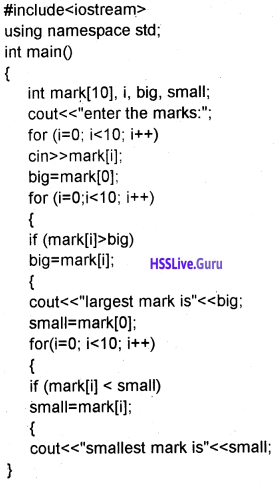Question 23.
Write the names of two searching methods in arrays. Prepare a chart that shows the comparisons of two searching methods. (MARCH-2015)
It is the process of finding the position of the given element.
1. Linear search:
In this method each element of the array is compared with the element to be searched starting from the first element. If it finds the position of the element in the array is returned.

2. Binary search:
It uses a technique called divide and conquer method. It can be performed only on sorted arrays. First we check the element with the middle element. There are 3 possibilities. The first possibility is the searched element is the middle element then search can be finished.

The second possibility is the element is less than the middle value so the upper bound is the middle element. The third possibility is the element is greater than the middle value so the lower bound is the middle element. Repeat this process.

Question 24.
Write a C++ program to illustrate array traversal. (SAY-2015 (IMP) (3)
#include<iostream>
using namespace std;
int main()
{
int i, mark;
for(i=0;i<50; i++)
{
cout<<“Enter mark “<<i + 1<<“: cirt>>mark[i];
}
cout<<“\nThe mark of 50 students after adding bonus mark 10 is given below \n”; for(i=0;i<50; i++)
cout<<mark[i] + 10<<endl;
}

Question 25.
Define an array. Also write an algorithm for searching an element in the array using any one method that you are familiar with. (MARCH-2016) (3)
An array is a collection of elements with same data type. The index of first element is 0 (zero) and the index of last element is size -1. There are 2 methods linear search and binary search.

Searching: It is the process of finding the position of the given element.
1. Linear search:
In this method each element of the array is compared with the element to be searched starting from the first element. If it finds the position of the element in the array is returned.

2. Binary search:
It uses a technique called divide and conquer method. It can be performed only on sorted arrays. First we check the element with the middle element. There are 3 possibilities. The first possibility is the searched element is the middle element then search can be finished.

The second possibility is the element is less than the middle value so the upper bound is the middle element. The third possibility is the element is greater than the middle value so the lower bound is the middle element. Repeat this process.8.3 Two dimensional (2D) arrays.

Some occasions we have to store 6 different marks of 50 students. For this we use 2D arrays. An array with two subscripts is used, eg. int mark[r][c]; Here r is the row and c is the column.

Question 26.

1. Finding sum of all elements in an array is an example of _________ operation.
2. If 24, 45, 98, 56, 76, 24, 15 are the elements of an array, illustrate the working of selection sort for arranging the elements in descending order. (SCERT SAMPLE -1) (3)

1. Traversal

2. In selection sort the array is divided into two parts the sorted part and unsorted part. First smallest elemant in the unsorted part in searched and exchanged with the first elemant now there is 2 parts sorted part and unsorted part. This process continues.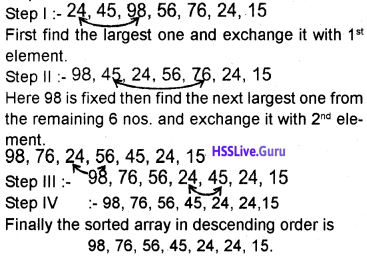Question 27.

1. How many bytes will be allocated for this array?
2. Write algorithm to sort this array using bubble sort method. (SCERT SAMPLE – II) (3)

1. To store an integer 4 bytes needed so AR needs 10 × 4 = 40 bytes.

2. Sorting – Arranging elements of an array in an order(ascending or descending).
(a) Bubble sort:
It is a simple sorting method. In this sorting considering two adjascent elements if it is out of order, the elements are interchanged. After the first iteration the largest(in the case of ascending sorting) or smallest(in the case of descending sorting) will be the end of the array. This process continues.

(b) Selection sort:
In selection sort the array is divided into two parts, the sorted part and unsorted part. First smallest element in the unsorted part is searched and exchanged with the first element. Now there is 2 parts sorted part and unsorted part. This process continues.Question 28.
Explain ‘Call by Value’ and ‘Call by Reference’ methods of function calling with the help of a suitable example. (SEP-2016) (3)
Two types are call by value and call by reference.
1. Call by value:
In call by value method the copy of the original value is passed to the function, if the function makes any change will not affect the original value.
Example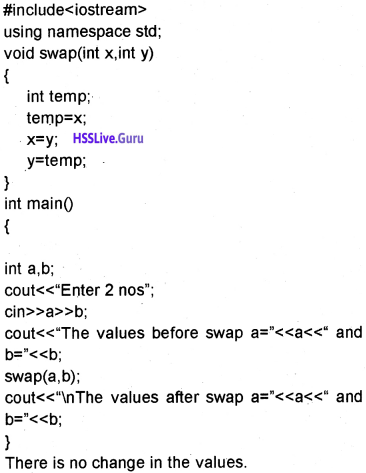2. Call by reference:
In call by reference method the address of the original value is passed to the function, if the function makes any change will affect the original value.
ExampleQuestion 29.
What is an array? Write C++ program to declare and use a single dimensional array for storing the computer science marks of all students in your
class. (SEP-2016) (3)
An array is a collection of elements with same data type or with the same name we can store many elements, the first or second or third etc can be distinguished by using the index(subscript). The first element’s index is 0, the second elements index is 1, and so on.Question 30.
Write an algorithm for arranging elements of an array in ascending order using bubble sort. (MARCH-2017) (3)